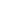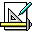Search found 4 results.Mathcad (1989)6 Mathematics Windows

Mathcad is computer software primarily intended for the verification, validation, documentation and re-use of engineering calculations.Mathematica (1994)4 Mathematics Windows

Mathematica, from Wolfram Research, Inc., is a mathematical and scientific application to formulate complex equations. and Mathworks MatLab.MathType (1990)5 Publishing Mathematics Windows

MathType is a tool for creating printable mathematical formulas. It includes a set of special fonts with mathematical symbols, and the application helps compose them. The results can then be copied in to word processors, or exported to graphics files. Editor.MatLab (1986)7 Mathematics Windows MacOS

MatLab is a high speed, interactive programming utility for manipulating, calculating, and plotting complicated mathematical equations. It is considered easy to use for those familiar with typical algebra equations.